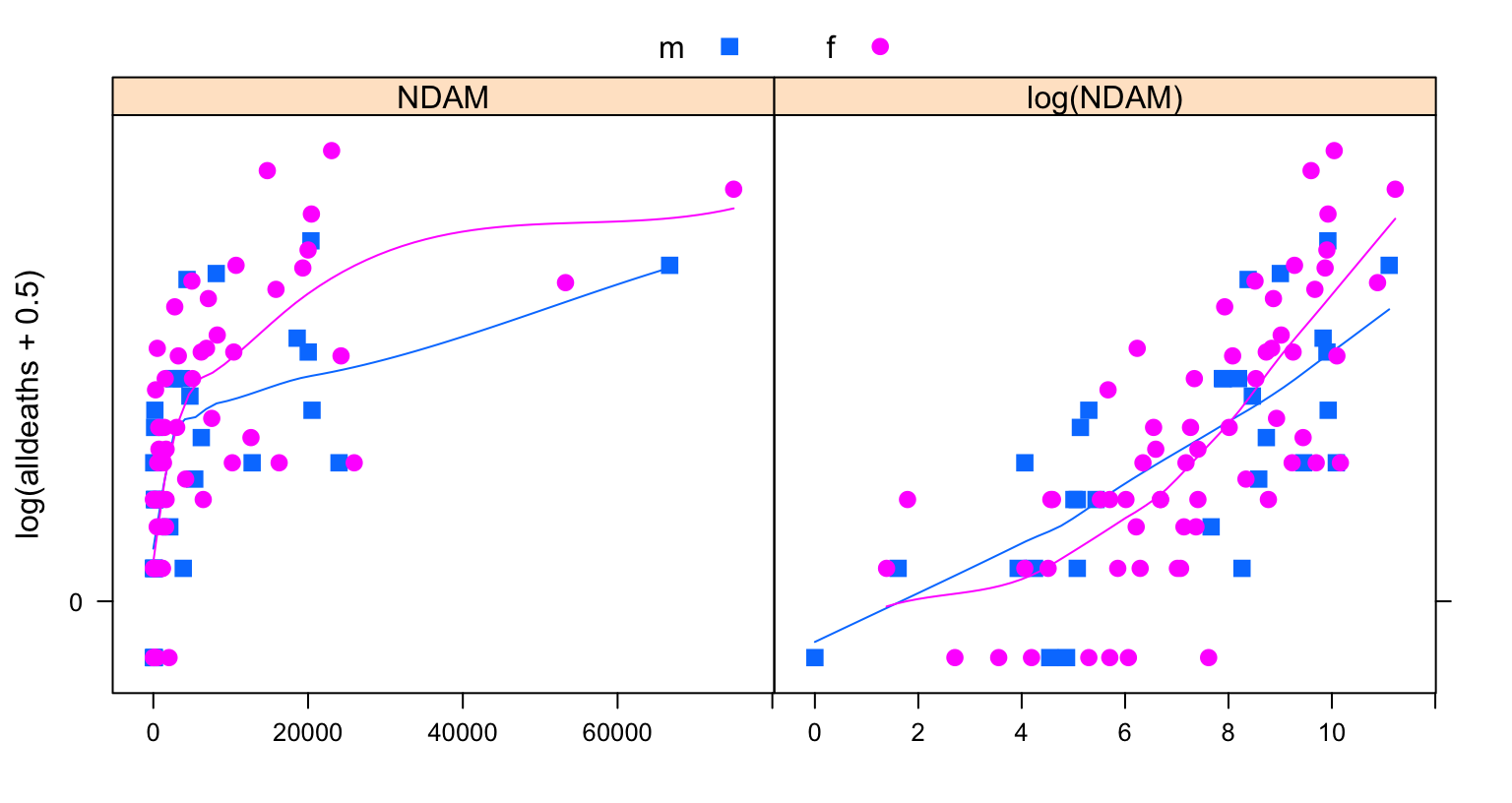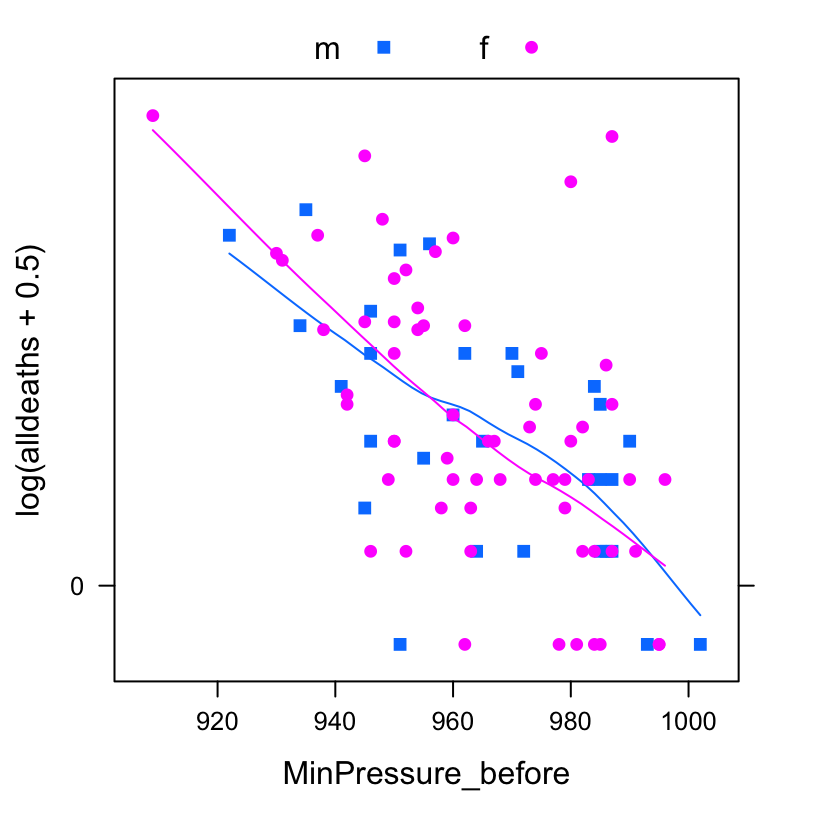# Female vs Male Hurricanes — Commentary

## John Maindonald

Dec 26 2014.

The notes that follow comment on analyses that are reported in Jung et al (2014), and in particular on the analysis that leads to their Figure 1. Diagnostic plots reveal serious problems with their analysis. Use of the Box-Cox methodology to identify a suitable power transformation leads to a model that fits the data well. It has `NDAM`\(^{0.13}\) as the only explanatary variable, with addition of terms involving the pressure at landfall and the masculinity-feminity index giving no improvement to the fit. Diagnostics are very satisfactory, with no outliers or highly influential points identified. For the modeling reported here, highly crucial roles attach both to preliminary graphical exploration and to careful examination of model diagnostics.

A further analysis works with the updated ICAT damage estimates that are in 2014 US dollars, and investigates inclusion of Audrey and Katrina that were omitted from the Jung et al analysis. Also examined is the model fit to data from 1979 onwards, when male and female names were alternated.

A further comment is that the regression coefficients are not capable of sustaining the interpretation that Jung et al wish to place on them.

Aside from the main theme are comments on the variety of functions, in different R packages, that are available for the fitting of regression models for data with a negative binomial error.

The data for Audrey and Katrina is corrected relative to that used in the June 20 2014 version of this document.

### Preparatory Steps

``````fnam <- paste0("http://www.pnas.org/lookup/suppl/doi:10.1073/",
"pnas.1402786111/-/DCSupplemental/pnas.1402786111.sd01.xlsx")
if(!file.exists('hurricane-names.xlsx')){
}
library(xlsx)``````
``````Loading required package: rJava
``````hurricJ <- read.xlsx('~/r/_rn/Data/hurricane-names.xlsx',
sheetIndex=1, rowIndex=1:93)
rnam <- with(hurricJ, paste0(as.character(Name), Year))
row.names(hurricJ) <- rnam
hurricJ[,"Gender_MF"] <- factor(hurricJ[,"Gender_MF"], labels=c("m","f"))``````

#### Check variables

keyVar <- hurricJ[, c(“MasFem”,“alldeaths”,“MinPressure_before”,“Category”,“ZNDAM”,“Year”)] sapply(keyVar,mean)

#### Examine plots

Use a `log(alldeaths+0.5)` scale on the y-axis in order to separate points. Smooth curves have been added, separately for hurricanes with male and female names.

``````library(lattice)
ypos <- seq(from=0, to=200, by=50)
sc <- list(x=list(relation="free"), y=list(at=sqrt(ypos), labels=paste(ypos)))
xyplot(log(alldeaths+0.5) ~ NDAM+log(NDAM), groups=Gender_MF, xlab="",
par.settings=simpleTheme(pch=c(15,16),cex=1.1), data=hurricJ,
scale=sc, auto.key=list(columns=2), type=c('p',"smooth"))``````Use of a logarithmic x-axis scale ameliorates severe leverage issues. It also gives variables that are closer to linearly related. Curvsture in the smooths, at least for female names, suggests that a logarithmic transformation may be too extreme.

Now check the range of values of `MinPressure_before`.

``with(hurricJ, range(MinPressure_before))``
``  909 1002``

The ratio is 1.1 to 1, too small for a logarithmic or power transformation to make much difference.

Now (Figure 2) plot against `MinPressure_before`

``````xyplot(log(alldeaths+0.5) ~ MinPressure_before,
groups=Gender_MF, data=hurricJ,
par.settings=simpleTheme(pch=c(15,16)),
scale=sc, auto.key=list(columns=2), type=c('p',"smooth"))``````### Negative Binomial versus `lm` style Linear Models

Once the counts are large enough, there is little to distinguish a model that uses `log(alldeaths+0.5)` as the outcpme variabke from a Negative Binomial model that has `var[deaths]` proportional to `E[deaths]`\(^2\). For these data, with 41 of the counts 4 or less, the approximation is rough. It may nevertheless be useful for exploratory purposes.

The following fits the preferred Jung at al model.

``````library(MASS)
hurricJ0.nb <- glm.nb(alldeaths ~ 1, data=hurricJ)
hurricJ3.nb <- glm.nb(alldeaths ~
MasFem*(MinPressure_before+NDAM),
data=hurricJ)
## MasFem:NDAM indicates that the product of MasFem and NDAM
## is to be included as a variable in the model.``````

Now compare the two models.

``anova(hurricJ0.nb, hurricJ3.nb)``
``````Likelihood ratio tests of Negative Binomial Models

Response: alldeaths
Model theta Resid. df    2 x log-lik.
1                                    1 0.446        91            -705
2 MasFem * (MinPressure_before + NDAM) 0.811        86            -644
Test    df LR stat.  Pr(Chi)
1
2 1 vs 2     5     60.6 9.29e-12``````
``````## Pearson chi-squared for Jung et al preferred model
sum(resid(hurricJ3.nb, type='pearson')^2)/hurricJ3.nb[["df.residual"]]``````
`` 1.09``
``````##
## Check model coefficients
coef(summary(hurricJ3.nb))``````
``````                           Estimate Std. Error z value Pr(>|z|)
(Intercept)                7.13e+01   1.87e+01    3.81 1.37e-04
MasFem                    -6.16e+00   2.36e+00   -2.61 9.10e-03
MinPressure_before        -7.13e-02   1.92e-02   -3.71 2.09e-04
NDAM                      -4.78e-05   2.83e-05   -1.69 9.12e-02
MasFem:MinPressure_before  6.32e-03   2.43e-03    2.60 9.45e-03
MasFem:NDAM                1.69e-05   3.59e-06    4.70 2.62e-06``````

Figure 3 shows the diagnostic plots.

``````opar <- par(mar=c(3.5,3.5,2.1,0.6), mgp=c(2.25,0.5,0), mfrow=c(2,2))
plot(hurricJ3.nb)``````